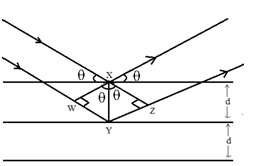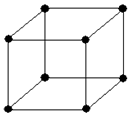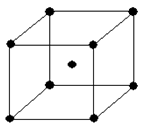Click to Chat

1800-1023-196

+91-120-4616500

CART 0

• 0

MY CART (5)

Use Coupon: CART20 and get 20% off on all online Study Material

ITEM
DETAILS
MRP
DISCOUNT
FINAL PRICE
Total Price: Rs.

There are no items in this cart.
Continue Shopping• Complete JEE Main/Advanced Course and Test Series
• OFFERED PRICE: Rs. 15,900
• View Details

Revision Notes on Solid State

Classification of solids:

Crystalline and  Amorphous solids:

S.No.

Crystalline Solids

Amorphous solids

1

Regular internal arrangement  of particles

irregular internal arrangement  of particles

2

Sharp melting point

Melt over a rage of temperature

3

Regarded as true solids

Regarded as super cooled liquids or pseudo solids

4

Undergo regular cut

Undergo irregular cut.

5

Anisotropic in nature

Isotropic in nature

Based on binding forces:

Crystal  Classification

Unit Particles

Binding Forces

Properties

Examples

Atomic

Atoms

London dispersion forces

Soft, very low melting, poor thermal and electrical conductors

Noble gases

Molecular

Polar or
non – polar molecules

Vander Waal’s forces (London dispersion, dipole – dipole forces hydrogen bonds)

Fairly soft, low to moderately high melting points, poor thermal and electrical conductors

Dry ice (solid, methane

Ionic

Positive and negative ions

Ionic bonds

Hard and brittle, high melting points, high heats of fusion, poor thermal and electrical conductors

NaCl, ZnS

Covalent

Atoms that are connected in covalent bond network

Covalent bonds

Very hard, very high melting points, poor thermal and electrical conductors

Diamond, quartz, silicon

Metallic Solids

Cations in electron cloud

Metallic bonds

Soft to very hard, low to very high melting points, excellent thermal and electrical conductors, malleable and ductile

All metallic elements, for example, Cu, Fe, Zn

Bragg Equation:

nλ = 2dsinθ,

Where

d= distance between the planes

n = order of refraction

θ= angel of refraction

λ = wavelengthCrystal Systems:

Total number of crystal systems: 7

Total number of Bravais Lattices: 14

Crystal Systems

Bravais Lattices

Intercepts

Crystal angle

Example

Cubic

Primitive, Face Centered, Body Centered

a = b = c

a = b = g = 90o

Pb,Hg,Ag,Au Diamond, NaCl, ZnS

Orthorhombic

Primitive, Face Centered, Body Centered, End Centered

a ≠ b ≠ c

a = b = g = 90o

KNO2, K2SO4

Tetragonal

Primitive, Body Centered

a = b ≠ c

a = b = g = 90o

TiO2,SnO2

Monoclinic

Primitive, End Centered

a ≠ b ≠ c

a = g = 90o, b≠ 90o

CaSO4,2H2O

Triclinic

Primitive

a ≠ b ≠ c

a≠b≠g≠900

K2Cr2O7, CaSO45H2O

Hexagonal

Primitive

a = b ≠ c

a = b = 900, g = 120o

Mg, SiO2, Zn, Cd

Rhombohedra

Primitive

a = b = c

a = g = 90o, b≠ 90o

As, Sb, Bi, CaCO3

Number of atoms in unit cells.

Primitive cubic unit cell:Number of atoms at corners = 8×1/8 =1

Number of atoms in faces = 0

Number of atoms at body-centre: =0

Total number of atoms = 1

Body-centred cubic unit cell:Number of atoms at corners = 8×1/8 =1

Number of atoms in faces = 0

Number of atoms at body-centre: =1

Total number of atoms = 2

Face-centred cubic or cubic-close packed unit cell:Number of atoms at corners = 8×1/8 =1

Number of atoms in faces = 6×1/2 = 3

Number of atoms at body-centre: = 0

Total number of atoms = 4

Packing Efficiency

Packing Efficiency = (Volume occupied by all the atoms present in unit cell / Total volume of unit cell)×100

Close structure

Number of atoms per unit cell ‘z’.

Relation between edge length ‘a’ and radius of atom ‘r’

Packing Efficiency

hcp and ccp or fcc

4

r = a/(2√2)

74%

bcc

2

r = (√3/4)a

68%

Simple cubic lattice

1

r = a/2

52.4%

Density of crystal lattice:

r = (Number of atoms per unit cell × Mass number)/(Volume of unit cell × NA)

or

Octahedral and Tetrahedral Voids:

Number of octahedral voids = Number of effective atoms present in unit cell

Number of tetrahedral voids = 2×Number of effective atoms present in unit cell

So, Number of tetrahedral voids = 2× Number of octahedral voids.

Coordination numbers

Geometry

Example

2

Linear

x < 0.155

BeF2

3

Planar Triangle

0.155 ≤ x < 0.225

AlCl3

4

Tetrahedron

0.225 ≤ x < 0.414

ZnS

4

Square planar

0.414 ≤ x < 0.732

PtCl42-

6

Octahedron

0.414 ≤ x < 0.732

NaCl

8

Body centered cubic

0.732 ≤ x < 0.999

CsCl

Classification of Ionic Structures:

Structures

Descriptions

Examples

Rock Salt Structure

Anion(Cl-) forms fcc units and cation(Na+) occupy octahedral voids. Z=4 Coordination number =6

NaCl, KCl, LiCl, RbCl

Zinc Blende Structure

Anion (S2-) forms fcc units and cation (Zn2+) occupy alternate tetrahedral voids Z=4 Coordination number =4

ZnS , BeO

Fluorite Structures

Cation (Ca2+) forms fcc units and anions (F-) occupy tetrahedral voids Z= 4 Coordination number of anion = 4 Coordination number of cation = 8

CaF2, UO2, and ThO2

Anti- Fluorite Structures

Oxide ions are face centered and metal ions occupy  all the tetrahedral voids.

Na2O, K2O and Rb2O.

Cesium Halide Structure

Halide  ions are primitive cubic while the metal ion occupies the center of the unit cell.
Z=2
Coordination number of = 8

All Halides of Cesium.

Pervoskite Structure

One of the cation is bivalent and the other is tetravalent. The bivalent ions are present in primitive cubic lattice with oxide ions on the centers of all the six square faces. The tetravalent cation is in the center of the unit cell occupying octahedral void.

CaTiO3, BaTiO3

Spinel and Inverse Spinel Structure

Spinel :M2+M23+O4, where M2+ is present in one-eighth of tetrahedral voids in a FCC lattice of oxide ions and M3+ ions are present in half of the octahedral voids. M2+ is usually Mg, Fe, Co, Ni, Zn and Mn;  M3+ is generally Al, Fe, Mn, Cr and Rh.

MgAl2O4 , ZnAl2O4, Fe3O4,FeCr2O4 etc.

Defects in crystal:

Stoichiometric Defects

1. Schottky Defects

Some of the lattice points in a crystal are unoccupied.

Appears in ionic compounds in which anions and cations are of nearly same size.

Decreases the density of lattice

Examples: NaCl and KCl

2.  Frenkel Defects

Ion dislocate from its position and occupies an interstitial position between the lattice points

Appears in crystals in which the negative ions are much larger than the positive ion.

Does not affect density of the crystal.

Examples: AgBr, ZnS

Non-Stoichiometric Defects

1. Metal Excess defect:

Metal excess defect occurs due to

anionic vacancies or

presence of extra cation.

F-Centres: hole produced due to absence of anion which is occupied by an electron.

2. Metal deficiency defect:

Metal deficiency defect occurs

due to variable valency of metals

when one of the positive ions is missing from its lattice site and the extra negative charge is balanced by some nearby metal ion acquiring two charges instead of one### Course Features

• 728 Video Lectures
• Revision Notes
• Previous Year Papers
• Mind Map
• Study Planner
• NCERT Solutions
• Discussion Forum
• Test paper with Video Solution